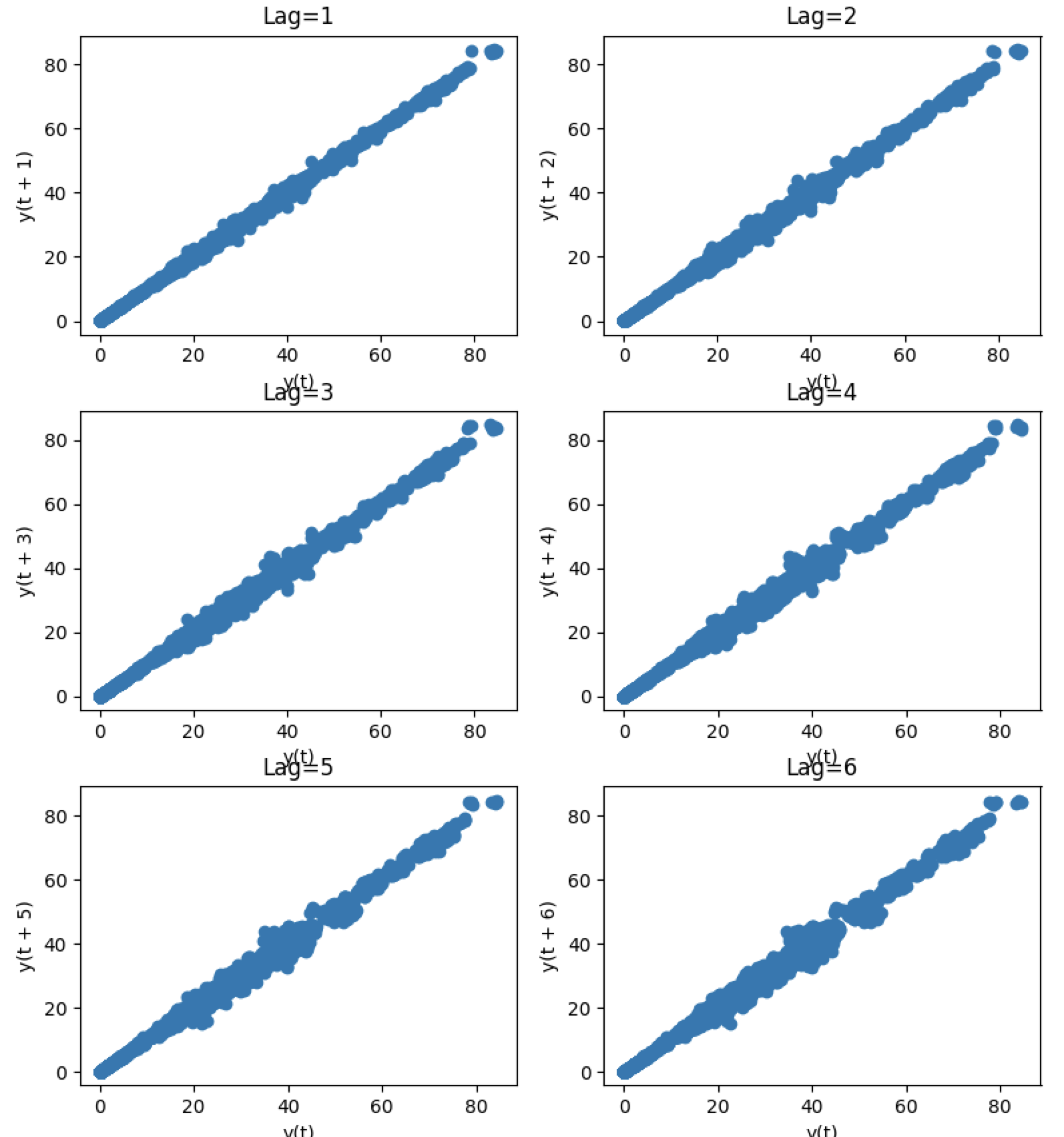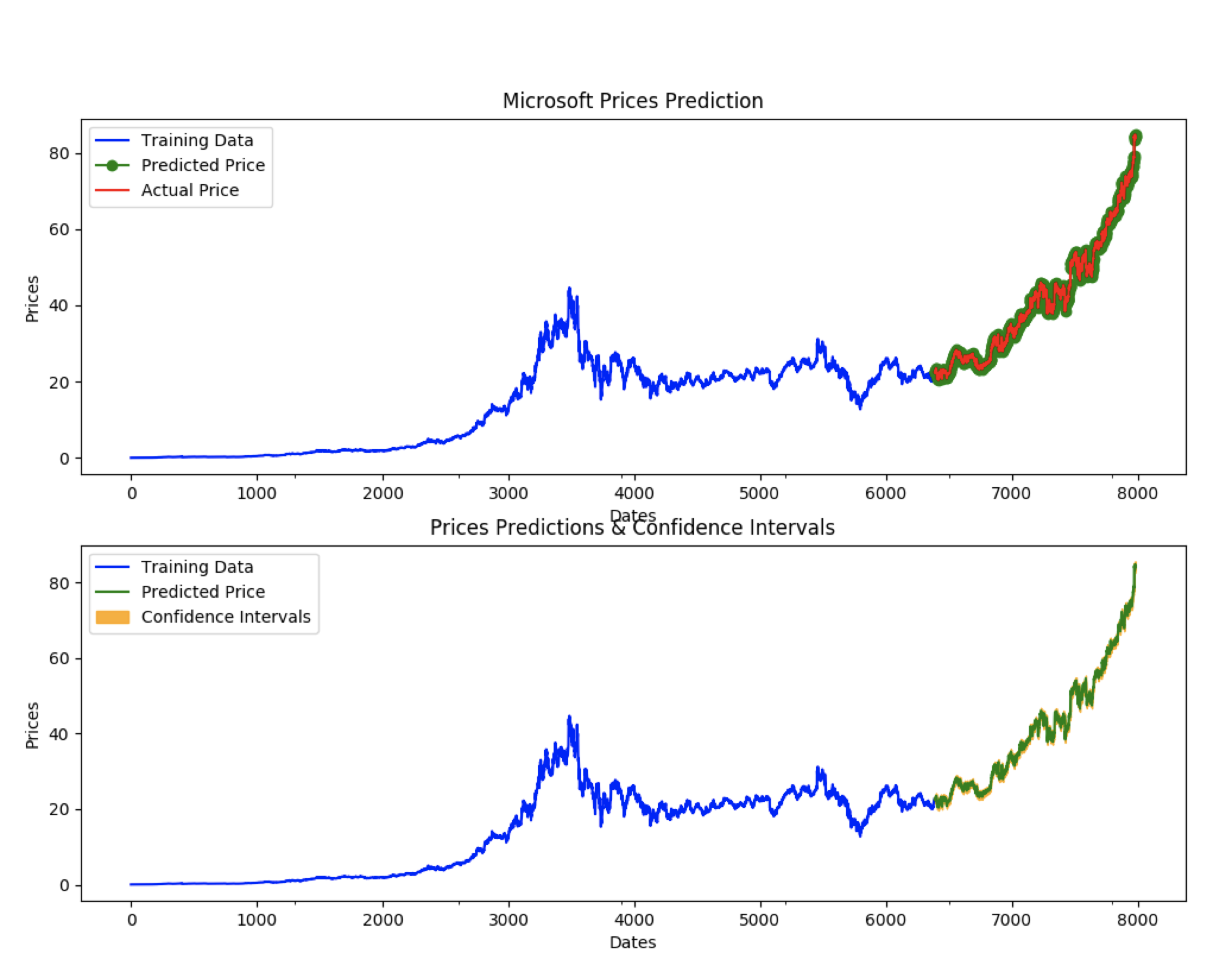## Stock Market Prediction

A recent post on Towards Data Science (TDS) demonstrated the use of ARIMA models to predict stock market data with raw statsmodels. This post addresses the same problem using pmdarima’s auto-ARIMA, and ends up achieving a different result with an even lower error rate.

Stock market analysis is always a hot topic, and it’s the place many young data scientists’ minds go to first when they enter the field. Therefore, I feel I must caveat this post with the warning that the stock market has been shown to be a random walk, and you should therefore take great care when attempting to forecast it via time series analysis. However, it makes for a fun exercise.

For this example, all we’ll need is Numpy, Pandas, pmdarima & Matplotlib. To run this example, you’ll need pmdarima version 1.5.2 or greater. If you’re running this in a notebook, make sure to include %matplotlib inline, or the plots will not show up under your cells!

This example was also designed for Python 3.6+. If you’re running python 3.5, simply remove the F-string print statements and it will work.

The pmdarima module conveniently includes the dataset we’ll be using as an internal utility. Rather than carting around .csv files, you can simply load the data from the package:

Date Open High Low Close Volume OpenInt
1986-03-13 0.06720 0.07533 0.06720 0.07533 1371330506 0
1986-03-14 0.07533 0.07533 0.07533 0.07533 409569463 0
1986-03-17 0.07533 0.07533 0.07533 0.07533 176995245 0

### Data splitting

As with all statistical and ML modeling, we need to make sure we’ve split our data so we can evaluate model performance on a hold-out set. However, unlike other traditional supervised learning, time series models intrinsically introduce endogenous temporality, meaning that the values at any given point $$y_{t}$$ in our time series likely have some effect on some future value, $$y_{t+n}$$. Therefore, we cannot simply split our data randomly; we must make a clean split in our time series (and exogenous variables, if present). Newer versions of pmdarima make this very simple using the train_test_split.

As in the TDS example, we’ll use 0.8 * dataSize as our training sample.

### Pre-modeling analysis

As you may know (if not, venture over to pmdarima’s tips-and-tricks doc before continuing), an ARIMA model has 3 core hyper-parameters, known as “order”:

• $$p$$: The order of the auto-regressive (AR) model (i.e., the number of lag observations)
• $$d$$: The degree of differencing.
• $$q$$: The order of the moving average (MA) model. This is essentially the size of the “window” function over your time series data.

Part of the science behind the auto-arima approach is intelligently finding the proper combination of $$p$$, $$d$$, and $$q$$ such that you achieve the best fit. The TDS article took the approach of fixing the $$p$$ parameter at 5 after examining auto-correlations with lag plots. A lag plot can provide clues about the underlying structure of your data:

• A linear shape to the plot suggests that an autoregressive model is probably a better choice.
• An elliptical plot suggests that the data comes from a single-cycle sinusoidal model.As you can see, all the lags look fairly linear, so it’s a good indicator that an auto-regressive model is a good choice. But since we don’t want to allow simple visual bias to impact our decision here, we’ll allow the auto_arima to select the proper lag term for us.

#### Estimating the differencing term

The TDS article selected $$d=1$$ as the differencing term. But how did they make that choice? With pmdarima, we can run several differencing tests against the time series to select the best number of differences such that the time series will be stationary.

Here, we’ll use the KPSS test and ADF test, selecting the maximum value between the two to be conservative. Fortunately, in this case, both tests indicated that $$d=1$$ was the best answer, but in the case where they disagreed, we could try both, selecting the best cross-validated result, or allow auto_arima to auto-select the $$d$$ term.

Therefore, we will use $$d=1$$.

### Fitting our model

Now it’s time to let the auto_arima method do its magic. First however, let’s examine some of the hyper parameters we’ll be setting:

• seasonal: If we were fitting a seasonal time series (of which the pmdarima package contains many: lynx, wineind & sunspots to name a few), we would set seasonal=True. This would also learn the $$P$$, $$D$$ and $$Q$$ hyper-parameters of the seasonal order. Fortunately, our time series is not seasonal in this example.

• stepwise: The stepwise algorithm will more intelligently select parameters for your ARIMA model, and tends to be faster than a random search. By default, this is true.

• suppress_warnings: MLE estimation can be noisy with warnings if something doesn’t converge. In our case, we just want to ignore the warnings.

• max_p: We can set our model to cap out at a certain value of $$p$$ or $$q$$. In this case, we’ll restrict the order of the AR model to be 6.

• trace: Controls the level of verbosity. 0 or False will not print, and 1 or above will print increasing levels of debug information.

Notice that we preset d=n_diffs, since we’ve already settled on a value for $$d$$. However, we’re allowing our ARIMA models explore various values of $$p$$ and $$q$$. After a few seconds, we arrive at the following solution:

Where the TDS model was of order (5, 1, 0), we ended up selecting a significantly more simple model. But how does it perform?

### Updating the model

Now that the heavy lifting of selecting model hyper-parameters has been performed, we can update our model by simulating days passing with our test set. For each new observation, we’ll let our model progress for several more iterations, allowing MLE to update its discovered parameters and shifting the latest observed value. Then we can measure the error on the forecasts. This can take a little while, since it’s relatively expensive to run several MLE steps and then evaluate a model repeatedly:

In the end, our model ended up way out-performing the TDS model!

Source MSE SMAPE
pmdarima 0.342 0.983 (!!)
TDS article 0.343 40.776

### Viewing forecasts

Let’s take a look at the forecasts our model produces overlaid on the actuals (in the first plot), and the confidence intervals of the forecasts (in the second plot):### Conclusion

The TDS article provided an awesome example of how to use ARIMAs to predict stocks. My hope in this example was to show how using pmdarima can simplify and enhance the models you build. If you’d like to check out the project, head over to its git repo. We’re always looking for new contributors!